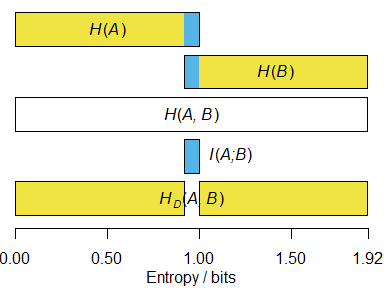# Comparing splits using information theory

#### 2020-09-17

To understand the information-based metrics implemented in ‘TreeDist’, it is useful to recall some basic concepts of information theory (see MacKay (2003) for an introduction).

## Shannon information

Information is usually measured in bits. One bit is the amount of information generated by tossing a fair coin: to record the outcome of a coin toss, I must record either a H or a T, and with each of the two symbols equally likely, there is no way to compress the results of multiple tosses.

The Shannon (1948) information content of an outcome $$x$$ is defined to be $$h(x) = -\log_2{P(x)}$$, which simplifies to $$\log_2{n}$$ when all $$n$$ outcomes are equiprobable. Thus, the information content of a fair coin toss is $$\log_2{2} = 1\textrm{ bit}$$; the information content of rolling a six-sided die is $$\log_2{6} \approx 2.58\textrm{ bits}$$; and the information content of selecting at random any one of the 105 unrooted binary six-leaf trees is $$\log_2{105} \approx 6.71\textrm{ bits}$$.

### Application to splits

The split $$S_1 =$$ AB|CDEF is found in 15 of the 105 six-leaf trees; as such, the probability that a randomly drawn tree contains $$S_1$$ is $$P(S_1) = \frac{15}{105}$$, and the information content $$h(S_1) = -\log_2{\frac{15}{105}} \approx 2.81\textrm{ bits}$$. Steel & Penny (2006) dub this quantity the phylogenetic information content.

Likewise, the split $$S_2 =$$ ABC|DEF occurs in nine six-leaf trees, so $$h(S_2) = -\log_2{\frac{9}{105}} \approx 3.54\textrm{ bits}$$. Three six-leaf trees contain both splits, so in combination the splits deliver $$h(S_1,S_2) = -\log_2{\frac{3}{105}} \approx 5.13\textrm{ bits}$$ of information.

Because $$h(S_1,S_2) < h(S_1) + h(S_2)$$, some of the information in $$S_1$$ is also present in $$S_2$$. The information in common between $$S_1$$ and $$S_2$$ is $$h_{shared}(S_1, S_2) = h(S_1) + h(S_2) - h(S_1,S_2) \approx 1.22\textrm{ bits}$$. The information unique to $$S_1$$ and $$S_2$$ is $$h_{different}(S_1,S_2) = 2h(S_1,S_2) - h(S_1) - h(S_2) \approx 3.91\textrm{ bits}$$.

These quantities can be calculated using functions in the ‘TreeTools’ package.

library('TreeTools', quietly = TRUE, warn.conflicts = FALSE)
library('TreeDist')
treesMatchingSplit <- c(
AB.CDEF = TreesMatchingSplit(2, 4),
ABC.DEF = TreesMatchingSplit(3, 3)
)
treesMatchingSplit
## AB.CDEF ABC.DEF
##      15       9
proportionMatchingSplit <- treesMatchingSplit / NUnrooted(6)
proportionMatchingSplit
##    AB.CDEF    ABC.DEF
## 0.14285714 0.08571429
splitInformation <- -log2(proportionMatchingSplit)
splitInformation
##  AB.CDEF  ABC.DEF
## 2.807355 3.544321
treesMatchingBoth <- TreesConsistentWithTwoSplits(6, 2, 3)
combinedInformation <- -log2(treesMatchingBoth / NUnrooted(6))

sharedInformation <- sum(splitInformation) - combinedInformation
sharedInformation
##  1.222392
# Or more concisely:
SplitSharedInformation(n = 6, 2, 3)
##  1.222392

## Entropy

Entropy is the average information content of each outcome, weighted by its probability: $$\sum{-p \log_2(p)}$$. Where all $$n$$ outcomes are equiprobable, this simplifies to $$\log_2{n}$$.

Consider a case in which Jane rolls a dice, and makes two true statements about the outcome $$x$$:

$$S_1$$: “Is the roll even?”.

• Two equally-possible outcomes: yes or no
• Entropy: $$H(S_1) = \log_2{2} = 1\textrm{ bit}$$.

$$S_2$$: “Is the roll greater than 3?”

• Two equally-possible outcomes: yes or no
• Entropy: $$H(S_2) = \log_2{2} = 1\textrm{ bit}$$.

The joint entropy of $$S_1$$ and $$S_2$$ is the entropy of the association matrix that considers each possible outcome:

$$S_1: x$$ odd $$S_1: x$$ even
$$S_2: x \le 3$$ $$x \in {1, 3}; p = \frac{2}{6}$$ $$x = 2; p = \frac{1}{6}$$
$$S_2: x > 3$$ $$x = 5; p = \frac{1}{6}$$ $$x \in {4, 6}; p = \frac{2}{6}$$

\begin{aligned} H(S_1, S_2) = \frac{2}{3}\log_2{\frac{2}{3}} + \frac{1}{3}\log_2{\frac{1}{3}} + \frac{1}{3}\log_2{\frac{1}{3}} + \frac{2}{3}\log_2{\frac{2}{3}} \approx 1.84 \textrm{ bits} \end{aligned}

Note that this less than the $$\log_2{6} \approx 2.58\textrm{ bits}$$ we require to determine the exact value of the roll: knowledge of $$S_1$$ and $$S_2$$ is not guaranteed to be sufficient to unambiguously identify $$x$$.

The mutual information between $$S_1$$ and $$S_2$$ describes how much knowledge of $$S_1$$ reduces our uncertainty in $$S_2$$ (or vice versa). So if we learn that $$S_1$$ is ‘even’, we become a little more confident that $$S_2$$ is ‘greater than three’.

The mutual information $$I(S_1;S_2)$$ corresponds to the sum of the individual entropies, minus the joint entropy:

\begin{aligned} I(S_1;S_2) = H(S_1) + H(S_2) - H(S_1, S_2) \end{aligned}

The entropy distance, also termed the variation of information (Meilă, 2007), is the information that $$S_1$$ and $$S_2$$ do not have in common:

\begin{aligned} H_D(S_1, S_2) = H(S_1, S_2) - I(S_1;S_2) = 2H(S_1, S_2) - H(S_1) - H(S_2) \end{aligned}### Application to splits

Let split $$S$$ divides $$n$$ leaves into two partitions $$A$$ and $$B$$. The probability that a randomly chosen leaf $$x$$ is in partition $$k$$ is $$P(x \in k) = \frac{|k|}{n}$$. $$S$$ thus corresponds to a random variable with entropy $$H(S) = -\frac{|A|}{n} \log_2{\frac{|A|}{n}} - \frac{|B|}{n}\log_2{\frac{|B|}{n}}$$ (Meilă, 2007).

The entropy of a split corresponds to the average number of bits necessary to transmit the partition label for a given leaf.

The joint entropy of two splits, $$S_1$$ and $$S_2$$, corresponds to the entropy of the association matrix of probabilities that a randomly selected leaf belongs to each pair of partitions:

$$S_1: x \in A_1$$ $$S_1: x \in B_1$$
$$S_2: x \in A_2$$ $$P(A_1,A_2) = \frac{|A_1 \cap A_2|}{n}$$ $$P(B_1,A_2) = \frac{|B_1 \cap A_2|}{n}$$
$$S_2: x \in B_2$$ $$P(A_1,B_2) = \frac{|A_1 \cap B_2|}{n}$$ $$P(B_1,B_2) = \frac{|B_1 \cap B_2|}{n}$$

$$H(S_1, S_2) = P(A_1,A_2) \log_2 {P(A_1,A_2)} + P(B_1,A_2) \log_2 {P(B_1,A_2)}$$

$$+ P(A_1,B_2)\log_2{P(A_1,B_2)} + P(B_1,B_2)\log_2{P(B_1,B_2)}$$

These values can then be substituted into the definitions of mutual information and entropy distance given above.

As $$S_1$$ and $$S_2$$ become more different, the disposition of $$S_1$$ gives less information about the configuration of $$S_2$$, and the mutual information decreases accordingly.